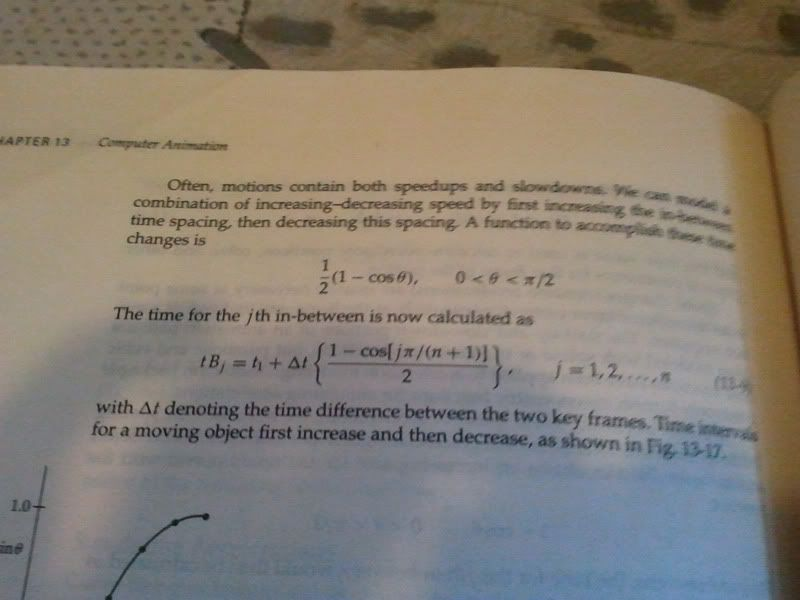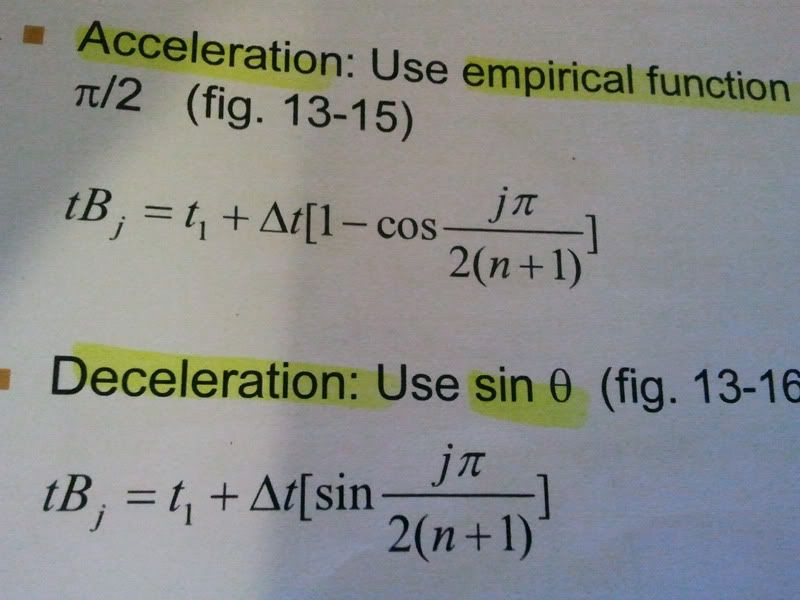# OpenGL Animation not work

Dear all, I’ve modified my previous opengl program which use while loop to perform the animation, due to high cpu usage, I modify the program using empirical functions to animate the robot arm, but outcome the arm does not move, is there any suggestions to correct my program so as to make it move ?Thanks for all your attentions

``````
//Import library
#include <stdlib.h>
#include <string.h>
#include <stdio.h>
#include <time.h>
#include <math.h>
#include <GL/glut.h>
#include "gsrc.h"

const double PI = 3.14159265;
// angles to rotate the base, lower and upper arms of the robot arm
double theta, phi, psi,th;
//time interval for the animation
long deltaT;
static GLfloat t1 = 0;
GLint j = 0;

//initialize the window and everything to prepare for display
void init_gl() {
//set the initial value of the 3 angles
theta = phi = psi = 0.0;
//set the time interval
deltaT = 10;
//set display color to white
glClearColor(1,1,1,0);
//clear and enable z-buffer
glClear (GL_DEPTH_BUFFER_BIT);
glEnable (GL_DEPTH_TEST);
//clear display window
glClear(GL_COLOR_BUFFER_BIT);
}

//Draw the base of the robot arm
void draw_base(){
glPushMatrix();
//to create the quadric objects

//set the color of the cylinder
glColor3f(1.0,0.0,0.0);
//Re-position the cylinder (x-z plane is the base)
glRotatef(-90,1.0,0.0,0.0);
//Draw the cylinder
gluCylinder(qobj, 30.0, 30.0, 40.0, 40.0, 40.0);
//Draw the upper disk of the base
gluDisk(qobju,0,30,40,40);

glPushMatrix();
//Change the M(lowdisk<updisk)
glTranslatef(0,0,40);
glColor3f(0,0,0);
//Draw the lower disk of the base
gluDisk(qobjl,0,30,40,40);
glPopMatrix();
glPopMatrix();
}

/****************************Texture Work Starts***************************************/
//Load the raw file for texture
/* Global Declarations */
#define IW	256				// Image Width
#define IH	256				// Image Height

//3D array to store image data
unsigned char InputImage     [IW][IH];

// Read an input image from a .raw file with double
void ReadRawImage ( unsigned char Image[][IH] )
{
FILE *fp;
int  i, j, k;
char* filename;
unsigned char temp;

filename = "floor.raw";

if ((fp = fopen (filename, "rb")) == NULL)
{
printf("Error (ReadImage) : Cannot read the file!!
");
exit(1);
}

for ( i=0; i<IW; i++)
{
for ( j=0; j<IH; j++)
{
for (k = 0; k < 3; k++)       // k = 0 is Red  k = 1 is Green K = 2 is Blue
{
fscanf(fp, "%c", &temp);
Image[i][j][k] = (unsigned char) temp;
}
Image[i][j] = (unsigned char) 0;         // alpha = 0.0
}
}
fclose(fp);

}

/****************************Texture Work Ends***************************************/

//Draw the 2x2x2 cube with center (0,1,0)
void cube(){
glPushMatrix();
glTranslatef(0,1,0);
glutSolidCube(2);
glPopMatrix();
}

//Draw the lower arm
void draw_lower_arm(){
glPushMatrix();
glScalef(15.0/2.0,70.0/2.0,15.0/2.0);//scale half is enough (some part is in the negative side)
cube();
glPopMatrix();
}

//Draw the upper arm
void draw_upper_arm(){
glPushMatrix();
glScalef(15.0/2.0,40.0/2.0,15.0/2.0);//scale half is enough (some part is in the negative side)
cube();
glPopMatrix();
}

void drawCoordinates(){
glBegin (GL_LINES);
glColor3f (1,0,0);
glVertex3f (0,0,0);
glVertex3f (300,0,0);

glColor3f (0,1,0);
glVertex3f (0,0,0);
glVertex3f (0,300,0);

glColor3f (0,0,1);
glVertex3f (0,0,0);
glVertex3f (0,0,300);
glEnd();
}

//Our drawing starts here
void showobj(void) {
//set the projection and perspective parameters/arguments
GLint viewport;
glGetIntegerv( GL_VIEWPORT, viewport );
glMatrixMode(GL_PROJECTION);
gluPerspective( 45, double(viewport)/viewport, 0.1, 1000 );
glMatrixMode(GL_MODELVIEW);
gluLookAt( 0, 0, 400, 0, 0, 0, 0,1,0 );

// get the rotation matrix from the rotation user-interface
glMultMatrixf( gsrc_getmo() );

//Clear the display and ready to show the robot arm
init_gl();

//Draw coordinates
drawCoordinates();

//Robot Drawing Starts
//Rotate the base by theta degrees
glRotatef(theta,0.0,1.0,0.0);
//Draw the base
draw_base();
//M(B<La)
glTranslatef(0.0,40.0,0.0);
//Rotate the lower arm by phi degree
glRotatef(phi,0.0,0.0,1.0);
//change the color of the lower arm
glColor3f(0.0,0.0,1.0);
//Draw the lower arm
draw_lower_arm();
//M(La<Ua)
glTranslatef(0.0,70.0,0.0);
//Rotate the upper arm by psi degree

glRotatef(psi,0.0,0.0,1.0);
//change the color of the upper arm
glColor3f(0.0,1.0,0.0);
//Draw the upper arm
draw_upper_arm();
//Drawing Finish
glutSwapBuffers();
glFlush ();
}

//To animate the robot arm
void animate(void)
{
th = j * PI / (2 * (10+1));
psi = t1 + deltaT * (1/2*(1-cos(th)));
j++;
if (j>10) j-=11;//reset j
glutPostRedisplay ();
}

//Deal with shadow matrix

}

void main (int argc, char** argv)
{
glutInit(&argc, argv);
//DOUBLE mode better for animation
// Set display mode.
glutInitDisplayMode( GLUT_DOUBLE | GLUT_RGB |GLUT_DEPTH);

glutInitWindowPosition( 50, 100 ); // Set top-left display-window position.
glutInitWindowSize( 400, 300 ); // Set display-window width and height.
glutCreateWindow( "My first OpenGL Game" ); // Create display window.

// Register mouse-click and mouse-move glut callback functions
// for the rotation user-interface.
//Allow user to drag the mouse and view the object
glutMouseFunc( gsrc_mousebutton );
glutMotionFunc( gsrc_mousemove );

// Display everything in showobj function
glutDisplayFunc(showobj);
//Perform background processing tasks or continuous animation
glutIdleFunc(animate);
glutMainLoop();
}

``````

A lot of issues.

1. The angle incremented in the idle callback is ‘th’ while a different variable ‘theta’ is used in the rotate function.
2. You are calling initGL in the render function that resets your angle theta to 0.
3. The condition on j in the idle callback prematurely stops the rotation. Just comment that condition.

See if this helps.

Thanks mobeen, item 2 has resolved but about the th value, I am trying to change the value of psi in this function >void animate(void), so that the upper arm can move, and I am following the textbook’s equation in order to simulate accelerate and deceleration, and according to the textbook, I need to change the value of j time by time to make it move, then why do I need to remove variable j?Well it is not that the eq. does not work, it works however, the increments of j produce a very small change in the value of theta. So i asked u to remove the expression for theta or u multiply theta by a scalar value or simply use a different value for angle of rotation.

Thanks mobeen, actually I don’t understand how to plug in the variables in my program, as you can see in the image, I have both the acc and dece equation, I use gettime to get the t1 (as the starting time) and I set deltaT as 5s (as the whole arm’s action is 5s), but something doesn’t make sense is that when I use gettime to get the time value, it is a very large long variable, then when I add up the second chunk (i.e. the […] part), it will certainly cannot make the arm move as I am trying to add a very small value to a very relatively large value, so I do not quite understand how such kind of equation works in animating my robot arm:(No this is wrong. Usually, we would calc. deltaT by subtracting the current time value from the time value at the initialization. Moreover, timeGetTime returns the total msecs since the start of your system.

Lets say u have two vars startTime and endTime.
startTime is initialized at the initialization by doing something like this;

``````
startTime = timeGetTime();

``````

Next in the render function, you get the time value again.

``````
void render() {
endTime = timeGetTime();

...
}

``````

You deltaTime can then be given as

``````
deltaT = (endTime - startTime); //in msecs
float deltaTSecs = deltaT/1000.0f;  //in secs

``````

Thanks mobeen, I used

``````
startT = time (&start);

``````

and

``````
//get the end time
time (&end);
//calculate deltaT
dif = difftime (end, start);

``````

to get the deltaT, is my method still correct?

And I want to ask what exactly does “deltaT” represent?
And from the image shown, can I manually set the value n and j by myself?

It looks ok u can test it urself by looking at the deltaT value.
From the image, it seems this formula is for doing in betweeening so probably j is ur loop variable and n is the total in betweening steps.

Thanks Mobeen, but the idle function (i.e. the animation function) is being executed continuously, it seems weird to add a for loop to loop the j value in an already looping idle function:(

Don’t use glutIdleFunc, as said by its name, its purpose is for doing things while the cpu is idling. So, it might be not accurate enough. Use glutPostRedisplay at the end of the display func (here showObj) instead, and calculate your time at the beginning of this function.